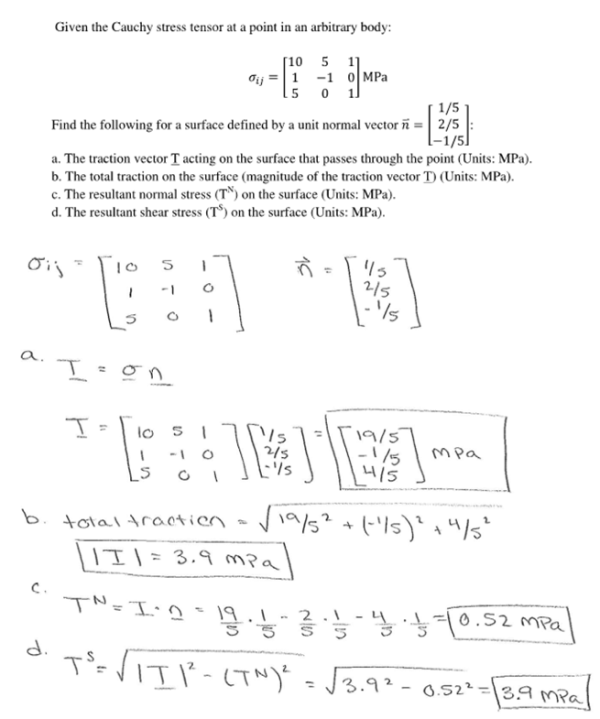Given the Cauchy stress tensor at a point in an arbitrary body, find the following for a surface defined by a unit normal vector n. a) The traction vector T acting on the surface that passes through the point. b) The total traction on the surface. c) The resultant normal stress on the surface. d) The resultant shear stress on the surface.Given the Cauchy stress tensor at a point in an arbitrary body, find the following for a surface defined by a unit normal vector n. a) The traction vector T acting on the surface that passes through the point. b) The total traction on the surface. c) The resultant normal stress on the surface. d) The resultant shear stress on the surface.

biomechanics page 1 biomechanics biomechanics biomechanics biomechanics biomechanics biomechanics biomechanics biomechanics page 2